Warning

This documents an unmaintained version of NetworkX. Please upgrade to a maintained version and see the current NetworkX documentation.

# square_clustering¶

square_clustering(G, nodes=None)[source]

Compute the squares clustering coefficient for nodes.

For each node return the fraction of possible squares that exist at the node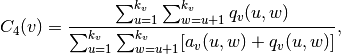where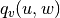are the number of common neighbors of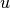and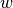other than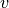(ie squares), and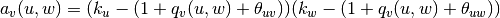, where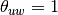ifandare connected and 0 otherwise.

Parameters: G (graph) – nodes (container of nodes, optional (default=all nodes in G)) – Compute clustering for nodes in this container. c4 – A dictionary keyed by node with the square clustering coefficient value. dictionary

Examples

>>> G=nx.complete_graph(5)
>>> print(nx.square_clustering(G,0))
1.0
>>> print(nx.square_clustering(G))
{0: 1.0, 1: 1.0, 2: 1.0, 3: 1.0, 4: 1.0}


Notes

While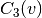(triangle clustering) gives the probability that two neighbors of node v are connected with each other,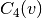is the probability that two neighbors of node v share a common neighbor different from v. This algorithm can be applied to both bipartite and unipartite networks.

References

  Pedro G. Lind, Marta C. González, and Hans J. Herrmann. 2005 Cycles and clustering in bipartite networks. Physical Review E (72) 056127.